# R Exercises and Solutions: ANOVA, Linear and Logistic Regression, Chi-Square Test

In order to solve the tasks you need:

## Problem 1

People who are concerned about their health may prefer hot dogs that are low in sodium and calories. The data file contains sample data on the sodium and calories contained in each of 54 major hot dog brands. The hot dogs are classified by type: beef (A), poultry (B), and meat (C).

The data file called hotdogs.rda contains the sodium and calorie content for random samples of each type of hot dog. This data set is included in the DS705data package.

### Part 1a

Make boxplots for the variable calories for the 3 types of hotdogs. Describe the 3 boxplots and the suitability of these samples for conducting analysis of variance.

load("hotdogs.rda")
boxplot(calories ~ type, data = hotdogs, xlab = "Hotdogs", ylab = "Calories")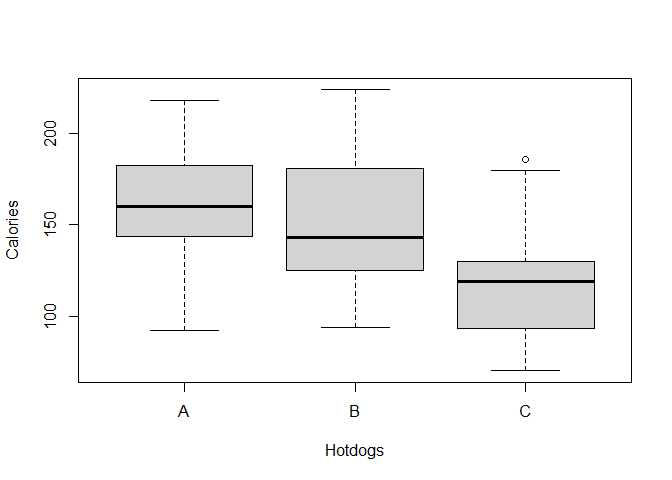The boxplot for hotdogs of type C is much lower than the boxplots for types A and B. This suggests that type C hotdogs are significantly different in terms of calories from the other two types of hotdogs. There is also an outlier for hotdogs of type C, which may lower the chance of rejecting the null hypothesis when conducting analysis of variance.

The distribution for type A is slightly skewed to the right, type B is also skewed to the right, and type C shows skewness to the left. Skewness, which is a sign of nonnormality also shows that the samples may not be very suitable for analysis of variance.

The length of the boxes for types A and C are very similar, while the box for type B is longer. This shows lack of homogeneity of variances, which is not suitable for analysis of variance.

### Part 1b

Conduct an analysis of variance test (the standard one that assumes normality and equal variance) to compare population mean calorie counts for these three types of hot dogs. (i) State the null and alternative hypotheses, (ii) use R to compute the test statistic and p-value, and (iii) write a conclusion in context at $$\alpha=0.10$$.

$$H_0: \mu_A = \mu_B = \mu_C$$

$$H_a:$$ Means are not all equal.

fit <- aov(calories ~ type, data = hotdogs)
summary(fit)
##             Df Sum Sq Mean Sq F value  Pr(>F)
## type         2  15425    7712   6.278 0.00365 **
## Residuals   51  62649    1228
## ---
## Signif. codes:  0 '***' 0.001 '**' 0.01 '*' 0.05 '.' 0.1 ' ' 1

Since p-value = 0.00365 < 0.1, we reject the null hypothesis. Some means are statistically significantly different. Population mean calorie counts for these three types of hot dogs are not the same.

### Part 1c

Follow up with the Tukey-Kramer multiple comparison procedure using a 90% experiment-wise confidence level.

1. Provide the R code used and

2. Write an interpretation for your multiple comparison output in the context of the problem; include the intervals for which population means are significantly different.

TukeyHSD(fit, conf.level = 0.90)
##   Tukey multiple comparisons of means
##     90% family-wise confidence level
##
## Fit: aov(formula = calories ~ type, data = hotdogs)
##
## $type ## diff lwr upr p adj ## B-A -9.144118 -33.41772 15.129490 0.7102899 ## C-A -39.673529 -63.94714 -15.399922 0.0033799 ## C-B -30.529412 -55.76791 -5.290917 0.0371484 This output indicates that the differences C-A and C-B are significant (p adj < 0.1), while B-A is not significant (p adj = 0.71 > 0.1). This means that type C hotdogs are significantly different in terms of population mean calorie counts from the other two types of hotdogs. The 90% confidence interval on the difference between means for types C and A extends from -63.947 to -15.400 and for types C and B - from -55.768 to -5.291. ### Part 1d As part of a vigorous road test for independent random samples of size 20 for 4 different brands of tires, the tread wear was measured for comparison. The data frame treadwear.rda contains the resulting data. Begin by exploring the sample means and standard deviations for each brand and looking at a boxplot comparison. That is, find the sample means and standard deviations and produce a boxplots of the tread wear measures for the four brands of tires. Conduct hypothesis tests for the normality of the tread wear for each brand using a 5% level of significance in each case. Also test for the homogeneity of the variances using $$\alpha=0.05$$. Comment on the results of each. ### Answer 1d load("treadwear.rda") tbl <- cbind(aggregate(wear ~ brand, data = treadwear, mean), aggregate(wear ~ brand, data = treadwear, sd)) colnames(tbl) <- c("brand", "wear.mean", "wear.sd") tbl ## brand wear.mean wear.sd ## 1 A 576.3017 148.10367 ## 2 B 671.1044 111.29454 ## 3 C 825.9870 57.85366 ## 4 D 853.2877 81.23036 boxplot(wear ~ brand, data = treadwear, xlab = "Brand", ylab = "Wear")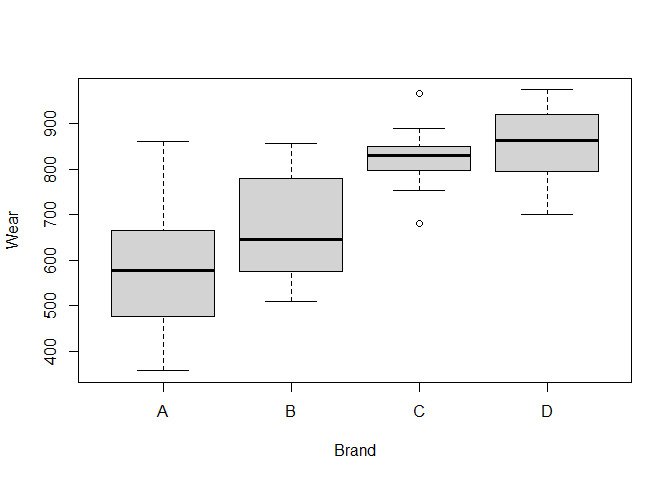# hypothesis tests for the normality shapiro.test(treadwear$wear[treadwear$brand == "A"]) ## ## Shapiro-Wilk normality test ## ## data: treadwear$wear[treadwear$brand == "A"] ## W = 0.94655, p-value = 0.3177 shapiro.test(treadwear$wear[treadwear$brand == "B"]) ## ## Shapiro-Wilk normality test ## ## data: treadwear$wear[treadwear$brand == "B"] ## W = 0.92027, p-value = 0.1003 shapiro.test(treadwear$wear[treadwear$brand == "C"]) ## ## Shapiro-Wilk normality test ## ## data: treadwear$wear[treadwear$brand == "C"] ## W = 0.95389, p-value = 0.4301 shapiro.test(treadwear$wear[treadwear$brand == "D"]) ## ## Shapiro-Wilk normality test ## ## data: treadwear$wear[treadwear$brand == "D"] ## W = 0.95129, p-value = 0.3871 # test for the homogeneity of the variances bartlett.test(wear ~ brand, data = treadwear) ## ## Bartlett test of homogeneity of variances ## ## data: wear by brand ## Bartlett's K-squared = 17.034, df = 3, p-value = 0.0006956 Results of Shapiro-Wilk normality test show that the p-value for each brand is larger than $$\alpha$$ (0.05) so the null hypothesis that the data came from a normally distributed population cannot be rejected. The tread wear for each brand is normally distributed. Results for Bartlett test of homogeneity of variances show that the p-value is smaller than $$\alpha$$ (0.0006956 < 0.05), which means that we reject the null hypothesis that the variances of tread wear for each of the brands are the same. At least one brand’s variance is statistically significantly different from the others. ### Part 1e What is the most appropriate inference procedure to compare population mean tread wear for these four brands of tires? Perform this procedure. 1. State the null and alternative hypotheses, (ii) use R to compute the test statistic and p-value, and (iii) write a conclusion in context at $$\alpha=0.05$$. ### Answer 1e Welch ANOVA (since variances are not equal). $$H_0: \mu_A = \mu_B = \mu_C$$ $$H_a:$$ Means are not all equal. oneway.test(wear ~ brand, data = treadwear) ## ## One-way analysis of means (not assuming equal variances) ## ## data: wear and brand ## F = 27.201, num df = 3.000, denom df = 40.197, p-value = 8.988e-10 Since p-value = 8.988e-10 < 0.05, we reject the null hypothesis. Some means are statistically significantly different. Population mean tread wear for these four brands of tires are not the same. ### Part 1f Conduct the most appropriate multiple comparisons procedure to determine which brands have significantly different tread wear. Use a family-wise error rate of $$\alpha=0.05$$. Use complete sentences to interpret the results in the context of the problem. ### Answer 1f library(userfriendlyscience) oneway(treadwear$wear, treadwear$brand, posthoc = 'games-howell') ## ### Oneway Anova for y=wear and x=brand (groups: A, B, C, D) ## ## Omega squared: 95% CI = [.38; .64], point estimate = .53 ## Eta Squared: 95% CI = [.41; .63], point estimate = .55 ## ## SS Df MS F p ## Between groups (error + effect) 1029881.43 3 343293.81 31.02 <.001 ## Within groups (error only) 841065.2 76 11066.65 ## ## ## ### Post hoc test: games-howell ## ## diff ci.lo ci.hi t df p ## B-A 94.80 -16.88 206.48 2.29 35.27 .120 ## C-A 249.69 151.80 347.57 7.02 24.67 <.001 ## D-A 276.99 174.18 379.79 7.33 29.48 <.001 ## C-B 154.88 78.40 231.37 5.52 28.57 <.001 ## D-B 182.18 99.07 265.30 5.91 34.77 <.001 ## D-C 27.30 -32.90 87.50 1.22 34.33 .616 This output indicates that the differences C-A, D-A, C-B and D-B are significant (p adj < 0.05), while B-A and D-C are not significant (p adj > 0.05). This means that brand C and brand D tires are significantly different in terms of population mean wear from brand A and brand B tires. ## Problem 2 This dataset contains the prices of ladies’ diamond rings and the carat size of their diamond stones. The rings are made with gold of 20 carats purity and are each mounted with a single diamond stone. The data was presented in a newspaper advertisement suggesting the use of simple linear regression to relate the prices of diamond rings to the carats of their diamond stones. The data is in the file diamond.rda and is included in the DS705data package. ### Part 2a Does it appear that a linear model is at least possibly a plausible model for predicting the price from carats of the diamonds for these rings? Begin by creating a scatterplot and comment on the suitability of a linear regression model. ### Answer 2a load("diamond.rda") plot(diamond)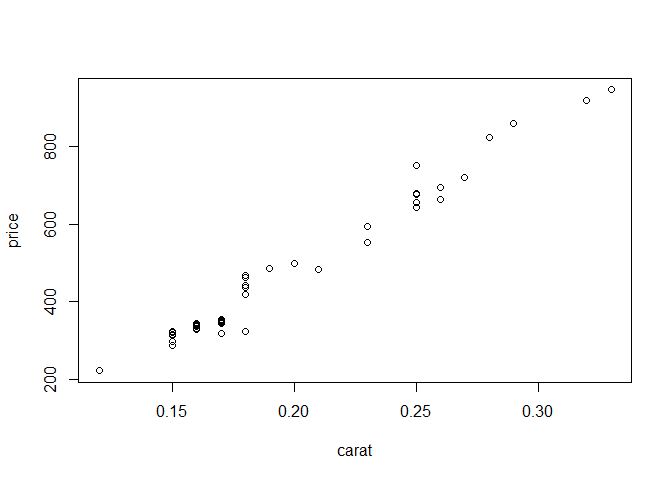A linear model looks like a plausible model for predicting the price from carats of the diamonds for these rings. ### Part 2b Obtain the estimated y-intercept and slope for the estimated regression equation and write the equation in the form price$$=\hat{\beta_0} + \hat{\beta_1}$$carats (only with $$\hat{\beta_0}$$ and $$\hat{\beta_1}$$ replaced with the numerical estimates from your R output). ### Answer 2b mod <- lm(price ~ carat, data = diamond) mod ## ## Call: ## lm(formula = price ~ carat, data = diamond) ## ## Coefficients: ## (Intercept) carat ## -250.6 3671.4 Replace the ## symbols with your slope and intercept $$\widehat{price}$$ = -250.6 + 3671.4 carat ### Part 2c Compute the sample Pearson correlation coefficient and test whether or not the population Pearson correlation coefficient between price and carat is zero using a 1% level of significance. (i) State the null and alternative hypotheses, (ii) test statistic, (iii) the p-value, and (iv) conclusion. ### Answer 2c cor(diamond$carat, diamond$price) ##  0.9875512 cor.test(diamond$carat, diamond$price, conf.level = 0.99) ## ## Pearson's product-moment correlation ## ## data: diamond$carat and diamond$price ## t = 42.116, df = 45, p-value < 2.2e-16 ## alternative hypothesis: true correlation is not equal to 0 ## 99 percent confidence interval: ## 0.9731306 0.9942549 ## sample estimates: ## cor ## 0.9875512 Sample correlation: 0.988 $$H_0:$$ True correlation is 0 $$H_a:$$ True correlation is not equal to 0 t = 42.116 p-value < 2.2e-16 Since p-value < 0.01, we reject the null hypothesis. True correlation between price and carat is statistically significantly different from zero. ### Part 2d Provide a 95% confidence interval to estimate the slope of the regression equation and interpret the interval in the context of the application (do not us the word “slope” in your interpretation). ### Answer 2d confint(mod, "carat", level = 0.95) ## 2.5 % 97.5 % ## carat 3495.818 3846.975 The 95% confidence interval for slope of the regression equation is (3495.818, 3846.975). It means that 95% of the time, if the carats of diamond stones increase by 0.01, the increase in the expected price of diamond ring is between 34.95818 and 38.46975. ### Part 2e Check to see if the linear regression model assumptions are reasonable for this data. (Step 1) Are the residuals normal? Construct a histogram, normal probability plot, and boxplot of the residuals and perform a Shapiro-Wilk test for normality. ### Answer 2e.1 res <- residuals(mod) par(mfrow = c(2, 2)) hist(res) qqnorm(res) qqline(res) boxplot(res) par(mfrow = c(1, 1))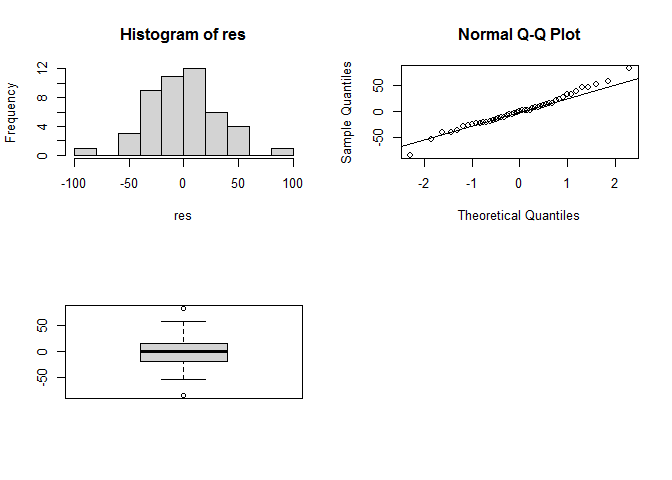shapiro.test(res) ## ## Shapiro-Wilk normality test ## ## data: res ## W = 0.98604, p-value = 0.8406 All of the above plots indicate that the residuals are normally distributed. Also, since p-value in the Shapiro-Wilk normality test is higher than 0.05, the null hypothesis that the data came from a normally distributed population cannot be rejected. The residuals are normally distributed. (Step 2) Plot the residuals against the fitted values. Does the equal variances assumption seem reasonable? Does the linear regression line seem to be a good fit? ### Answer 2e.2 plot(diamond$carat, res,
ylab = "Residuals", xlab = "Fitted Values", main = "Residuals vs. Fitted")
abline(0, 0)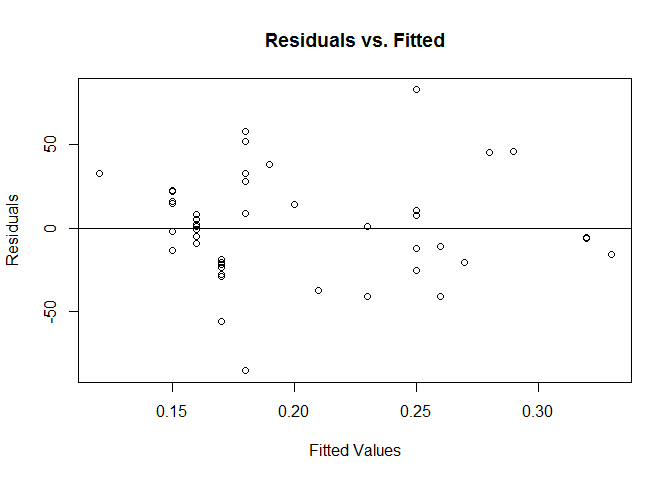The equal variances assumption seem reasonable and the regression line appears to be a good fit, because the residuals are equally spread around a horizontal line without distinct patterns.

(Step 3) Perform the Breusch-Pagan test for equal variances of the residuals. What does the test tell you?

library(lmtest)
bptest(mod)
##
##  studentized Breusch-Pagan test
##
## data:  mod
## BP = 0.18208, df = 1, p-value = 0.6696

p-value = 0.6696 > 0.05, so the null hypothesis of homoscedasticity cannot be rejected. Variances of the residuals are statistically significantly equal.

### Part 2f

Calculate and interpret the coefficient of determination $$r^2_{yx}$$ (same as $$R^2$$).

cor(diamond$carat, diamond$price)^2
##  0.9752573

The coefficient of determination shows that the model explains about 97.5% of variability of the price data around its mean.

### Part 2g

Should the regression equation obtained for price and carats be used for making predictions? Explain your answer.

The regression equation obtained for price and carats can be used for making predictions, since the coefficient of determination shows that the model fits the data very well and also the residuals’ analysis indicates that the model assumptions are satisfied.

### Part 2h

What would be the straightforward interpretation of the y-intercept in this regression model? Does it make sense here? Why would this not be appropriate as a stand-alone interpretation for this scenario? (hint: what is extrapolation?)

The y-intercept in this regression model would mean that the price for a ring with no diamond stone would be -250.6. It does not make sense, because the price cannot be a negative number. Also, this would not be appropriate as a stand-alone interpretation for this scenario because we cannot make any assumptions on how much a ring with no diamond would cost as it is beyond our observation range.

### Part 2i

Create 95% prediction and confidence limits for the population mean price for the carats given in the sample data and plot them along with a scatterplot of the data.

# 95% prediction limits
carat <- sort(diamond$carat) pred.int <- predict(mod, newdata = data.frame(carat = carat), interval = "prediction") # 95% confidence limits conf.int <- predict(mod, newdata = data.frame(carat = carat), interval = "confidence") # scatterplot plot(price ~ carat, data = diamond) # add prediction interval lines(carat, pred.int[, 2], col = "blue") lines(carat, pred.int[, 3], col = "blue") # add confidence interval lines(carat, conf.int[, 2], col = "red", lty = 2) lines(carat, conf.int[, 3], col = "red", lty = 2) # add legend legend("topleft", c("95% Confidence Interval", "95% Prediction Interval"), col = c("red", "blue"), lty = c(2, 1))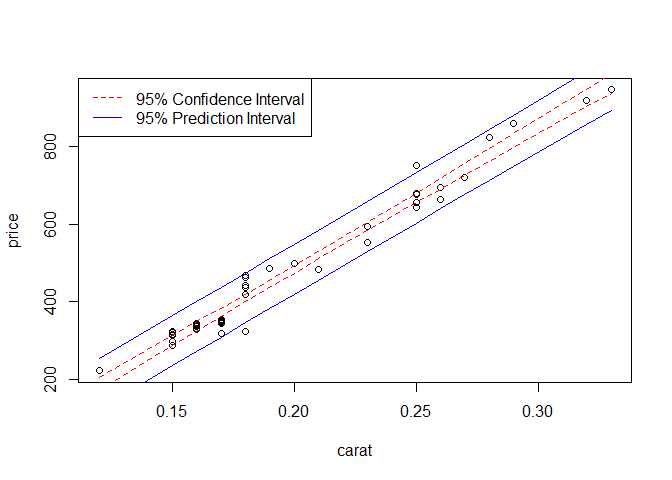## Problem 3 Blood type is classified as “A, B, or AB”, or O. In addition, blood can be classified as Rh+ or Rh -. In a survey of 500 randomly selected individuals, a phlebotomist obtained the results shown in the table below. Rh FactorA, B, or ABOTotal Rh+226198424 Rh-463076 Total272228500 ### Part 3a Conduct the appropriate test of significance to test the following research question “Is Rh factor associated with blood type?” Use a 5% level of significance and include all parts of the test. 1. Provide R code and state the following: 1. null and alternative hypotheses 2. test statistic 3. degrees of freedom 4. p-value (report to 4 decimal places, enter 0 if P < 0.00005) 5. conclusions. ### Answer 3a bloodtype <- matrix(c(226, 198, 46, 30), nrow = 2, byrow = TRUE) rownames(bloodtype) <- c("Rh+", "Rh-") colnames(bloodtype) <- c("A/B/AB", "O") bloodtype ## A/B/AB O ## Rh+ 226 198 ## Rh- 46 30 chisq.test(bloodtype) ## ## Pearson's Chi-squared test with Yates' continuity correction ## ## data: bloodtype ## X-squared = 1.0804, df = 1, p-value = 0.2986 $$H_0:$$ Rh factor and blood type are independent variables; $$H_a:$$ Rh factor and blood type are not independent. X-squared = 1.0804 df = 1 p-value = 0.2986 Since p-value = 0.2986 > 0.05, we cannot reject the null hypothesis that Rh factor and blood type are independent. Rh factor is not associated with blood type. ### Part 3b Compute and interpret the odds ratio of having Type O blood for Rh+ compared to Rh-. ### Answer 3b library(mosaic) oddsRatio(bloodtype) ##  1.343363 For Rh+, the odds of having Type O blood are 1.34 times larger than the odds for having Type O blood when blood is Rh-. ### Part 3c Construct and interpret a 90% confidence interval for the population proportion of people who are Rh-, given that they have Type O blood. ### Answer 3c n <- sum(bloodtype[, "O"]) k <- bloodtype["Rh-", "O"] # proportion point estimate pbar <- k/n pbar ##  0.1315789 # standard error SE <- sqrt(pbar ∗ (1 − pbar) / n) # margin of error E <- qnorm(0.95) ∗ SE # confidence interval pbar + c(−E, E) ##  0.09475603 0.16840187 At 90% confidence level, between 9.48% and 16.84% of people who have Type O blood are Rh-. ## Problem 4 The carinate dove shell is a yellowish to brownish colored smooth shell found along shallow water coastal areas in California and Mexico. A study was conducted to determine if the shell height of the carinate dove shell could be accurately predicted and to identify the independent variables needed to do so. Data was collected for a random sample of 30 of these gastropods and 8 variables that researchers thought might be good predictors were recorded. The shell heights (in mm) are labeled in the file as Y and the potential predictor variables are simply named as X1, X2, X3, X4, X5, X6, X7, and X8. Independent variables X1 through X7 are quantitative while X8 is categorical. The data is in the file shells.rda and is included in the DS705data package. ### Part 4a Use stepwise model selection with AIC as the stepping criterion and direction = “both” to identify the best first-order model for predicting shell height (Y). Identify the predictor variables in the final model as well as the AIC for that model. ### Answer 4a load("shells.rda") null <- lm(Y ~ 1, data = shells) full <- lm(Y ~ ., data = shells) mod_A <- step(null, scope = list(upper = full), data = shells, direction = "both", trace = FALSE) mod_A ## ## Call: ## lm(formula = Y ~ X2 + X4 + X1 + X6 + X7, data = shells) ## ## Coefficients: ## (Intercept) X2 X4 X1 X6 X7 ## 1.42718 0.65317 0.60923 1.15023 -0.06487 0.02636 extractAIC(mod_A) ##  6.0000 -121.9933 The predictor variables in the final model are X1, X2, X4, X6 and X7. The AIC for this model is -121.99. ### Part 4b Compute the variance inflation factor for the final model from part 4a. Does this model suffer from multicollinearity? Explain your answer. ### Answer 4b library(car) vif(mod_A) ## X2 X4 X1 X6 X7 ## 3.804041 2.697711 4.286121 4.428297 3.172575 This model does not suffer from multicollinearity, because VIF values are quite small and none of the VIF values exceed 10. ### Part 4c Let’s define Model B as follows: Y = $$\beta_0 + \beta_1 X_1 + \beta_2 X_2 + \beta_3 X_2^2 + \beta_4 X_4 + \beta_5 X_6 +\epsilon$$ Fit Model B and compare the AIC of it to the model that the stepwise selection procedure identified as best in 4a, which you may refer to as Model A. Which model is the better fitting model according to AIC? Explain your answer. ### Answer 4c mod_B <- lm(Y ~ X1 + X2 + I(X2^2) + X4 + X6, data = shells) mod_B ## ## Call: ## lm(formula = Y ~ X1 + X2 + I(X2^2) + X4 + X6, data = shells) ## ## Coefficients: ## (Intercept) X1 X2 I(X2^2) X4 X6 ## 9.16461 1.11286 -5.45450 1.14091 0.79526 -0.03961 extractAIC(mod_B) ##  6.0000 -129.4831 extractAIC(mod_A) ##  6.0000 -121.9933 According to AIC, the better fitting model is model B because the AIC of it is smaller. ### Part 4d Compute the variance inflation factor for Model B from part 4c. Does this model suffer from multicollinearity? Explain your answer. ### Answer 4d vif(mod_B) ## X1 X2 I(X2^2) X4 X6 ## 4.195143 373.677887 369.491380 2.119951 2.309547 Model B suffers from multicollinearity because VIF values for X2 and X2^2 are very high (both exceed 10, which is a sign of multicollinearity). ### Part 4e Center the variable X2 and compute the quadratic term associated with it (call them cX2 and cx2sq, respectively). We’ll identify this as Model C: Y = $$\beta_0 + \beta_1 X_1 + \beta_2 cX_2 + \beta_3 cX_2^2 + \beta_4 X_4 + \beta_5 X_6 +\epsilon$$ Compute the variance inflation factor for Model C. Does this model suffer from multicollinearity? Explain your answer. ### Answer 4e cX2 <- shells$X2 - mean(shells$X2) cX2sq <- cX2^2 shells <- cbind(shells, cX2, cX2sq) mod_C <- lm(Y ~ X1 + cX2 + cX2sq + X4 + X6, data = shells) mod_C ## ## Call: ## lm(formula = Y ~ X1 + cX2 + cX2sq + X4 + X6, data = shells) ## ## Coefficients: ## (Intercept) X1 cX2 cX2sq X4 X6 ## 2.70477 1.11286 0.52081 1.14091 0.79526 -0.03961 vif(mod_C) ## X1 cX2 cX2sq X4 X6 ## 4.195143 3.765151 1.036090 2.119951 2.309547 Model C does not suffer from multicollinearity, because all VIF values are small and none of them exceed 10. ### Part 4f Compare the adjusted R-squared for Models A and C. Explain what adjusted R-squared measures and state which model is “better” according to this criterion. ### Answer 4f summary(mod_A)$adj.r.squared
##  0.9413233
cX2sq = (2.4 - mean(shells$X2))^2, X4 = 3, X6 = 48) predict(mod_C, newdata, interval = "prediction") ## fit lwr upr ## 1 7.136107 6.907404 7.36481 The 95% prediction interval of (6.907, 7.365) means that 95% of the time, the shell height for a randomly selected shell with $$X1=3.6, X2=2.4, X4=3.0, and X6=48$$ will be between 6.907 and 7.365. ## Problem 5 A study on the Primary News Source for Americans was conducted using a random sample of 115 Americans in 2015. The results are shown below. TVRadioNewspaperInternet Sample from 201538201542 Distribution in 199545%18%16%21% Conduct the hypothesis test to determine if the distribution of Primary News Source for Americans is the same in 2015 as it was in 1995. Use $$\alpha = 0.10$$. State your hypotheses, test statistic, df, p-value, and conclusions, including a practical conclusion in the context of the problem. ### Answer 5 x <- c(38, 20, 15, 42) p <- c(0.45, 0.18, 0.16, 0.21) chisq.test(x, p = p) ## ## Chi-squared test for given probabilities ## ## data: x ## X-squared = 17.499, df = 3, p-value = 0.000558 $$H_0: p_1 = 0.45, p_2 = 0.18, p_3 = 0.16, p_4 = 0.21$$ $$H_a:$$ at lest one equation is not true Test statistic is 17.499, df = 3, p-value = 0.000558. Since p-value < 0.1, we reject the null hypothesis. At least one proportion is statistically significantly different from expected proportion. The distribution of Primary News Source for Americans in 2015 has changed since 1995. ## Problem 6 In an effort to make better cheese, a company has a random sample of 30 cheese consumers taste 30 specially prepared pieces of Australian cheddar cheese (1 piece for each person). Each subject rated the taste of their piece of cheese as “acceptable” (coded as 1) or “not acceptable” (coded as 0). One variable measured was called ACETIC and was a quantitative variable ranging from 4.5 to 6.5 units. The other variable recorded was whether the person was a child (person=1) or an adult (person=2). The data file called cheese.rda. This data set is included in the DS705data package. ### Part 6a Fit the first order model for predicting whether or not the taste of the cheese is acceptable (i.e. acceptable=1) from the acetic value and also whether the person was a child or an adult. At a 5% level of significance, should either variable be dropped from the model? ### Answer 6a load("cheese.rda") mod1 <- glm(taste ~ acetic + person, family = binomial, data = cheese) summary(mod1) ## ## Call: ## glm(formula = taste ~ acetic + person, family = binomial, data = cheese) ## ## Deviance Residuals: ## Min 1Q Median 3Q Max ## -2.2245 -0.4998 -0.2002 0.3040 1.6066 ## ## Coefficients: ## Estimate Std. Error z value Pr(>|z|) ## (Intercept) -18.709 8.517 -2.197 0.0280 * ## acetic 2.787 1.412 1.975 0.0483 * ## personAdult 3.096 1.371 2.258 0.0239 * ## --- ## Signif. codes: 0 '***' 0.001 '**' 0.01 '*' 0.05 '.' 0.1 ' ' 1 ## ## (Dispersion parameter for binomial family taken to be 1) ## ## Null deviance: 34.795 on 29 degrees of freedom ## Residual deviance: 20.550 on 27 degrees of freedom ## AIC: 26.55 ## ## Number of Fisher Scoring iterations: 6 At a 5% level of significance, no variable should be dropped from the model. ### Part 6b Convert the estimated coefficient of acetic to an odds ratio and interpret it in the context of the problem. ### Answer 6b exp(coef(mod1)) ## acetic ## 16.23497 The odds for taste being acceptable multiply by 16.23497 for every one-unit increase in the amount of acetic. ### Part 6c Compute the predicted probability of a child finding the taste of the cheese acceptable when the value for acetic is 6. ### Answer 6c newdata1 <- data.frame(acetic = 6, person = "Child") predict(mod1, newdata1, type = "response") ## 1 ## 0.1206732 ### Part 6d Compute a 95% confidence interval for the predicted probability of a child finding the taste of the cheese acceptable when the value for acetic is 6. ### Answer 6d pred <- predict(mod1, newdata1, type = "link", se.fit = TRUE) invlink <- mod1[["family"]][["linkinv"]] z <- qnorm(1 - (1-0.95)/2) low.ci <- pred$fit - z * pred$se.fit up.ci <- pred$fit + z * pred$se.fit prediction <- invlink(pred$fit)
data.frame(prediction = prediction, low.ci = low.ci.response, up.ci = up.ci.response)
##   prediction     low.ci     up.ci
## 1  0.1206732 0.01591904 0.5379397